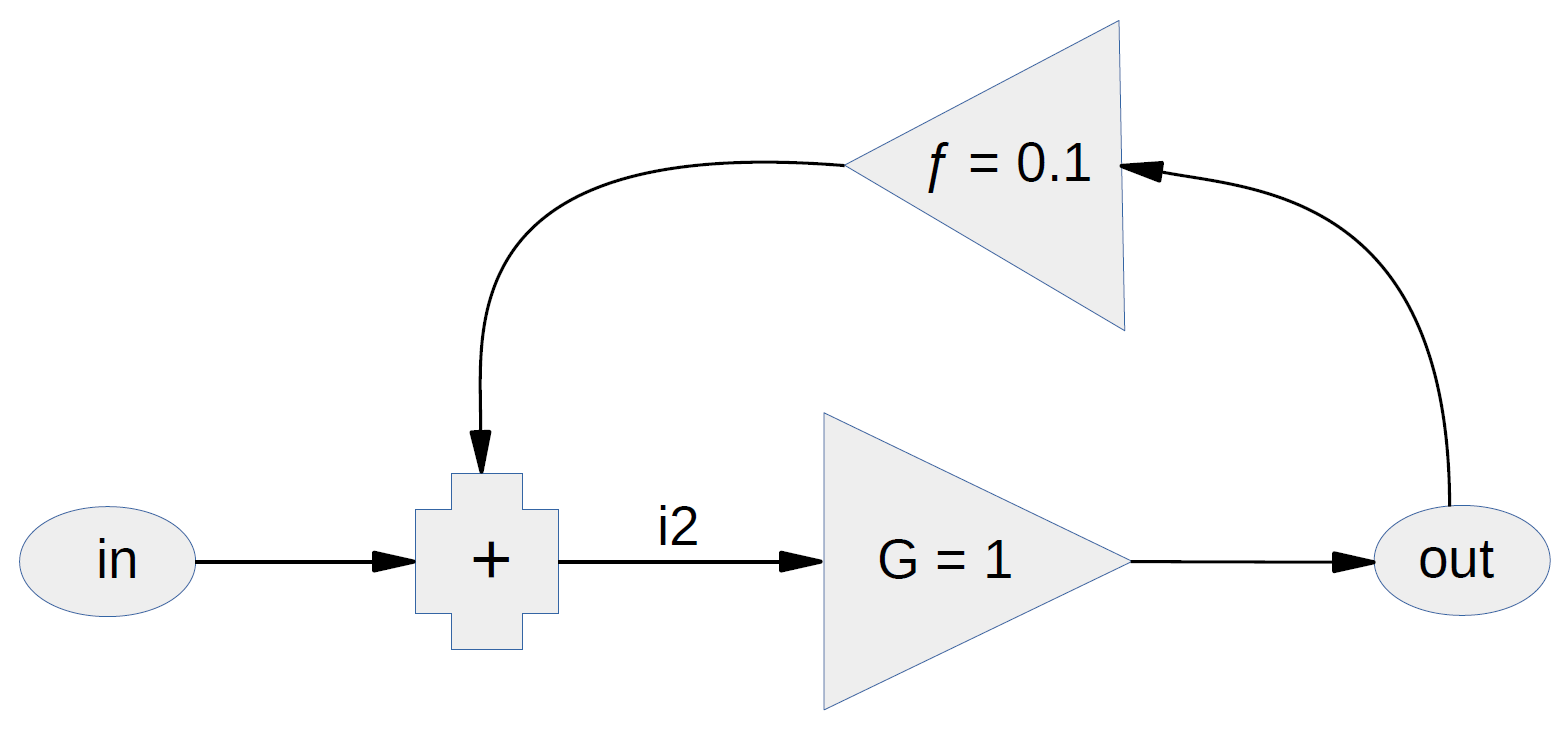﻿ Linear feedback system flow diagram

PDF version here: https://sealevel.info/linear_feedback_system_diagram1.pdf

# Linear feedback systemin = input
out = output
G = open-loop gain (assume 1.0 for simplicity)
ƒ = feedback (shown as +10%)

i2 = in + (ƒ × out)
out = i2 × G
= G × (in + (ƒ × out))
assume G = 1, then:
out = in + (ƒ × out)
out – (ƒ × out) = in
out × (1 – ƒ) = in
out = in / (1 × ƒ)

if ƒ = +10% then out = in / 0.9 = 1.11111… × in
if ƒ = +50% then out = in / 0.5 = 2 × in
if ƒ = 50% then out = in / 1.5 = 0.66666… × in